SLVSCM8D May   2015  – August 2021

PRODUCTION DATA

1. Features
2. Applications
3. Description
4. Revision History
5. Pin Configuration and Functions
6. Specifications
7. Detailed Description
1. 7.1 Overview
2. 7.2 Functional Block Diagram
3. 7.3 Feature Description
4. 7.4 Device Functional Modes
1. 7.4.1 Operation
8. Application and Implementation
1. 8.1 Application Information
2. 8.2 Typical Application
1. 8.2.1 Design Requirements
2. 8.2.2 Detailed Design Procedure
3. 8.2.3 Application Curves
9. Power Supply Recommendations
10. 10Layout
11. 11Device and Documentation Support
12. 12Mechanical, Packaging, and Orderable Information

• RHL|20
• RHL|20

#### 8.2.2.8 Loop Stability

The TPS61088 requires external compensation, which allows the loop response to be optimized for each application. The COMP pin is the output of the internal error amplifier. An external compensation network comprised of resistor R5, ceramic capacitors C5 and C8 is connected to the COMP pin.

The power stage small signal loop response of constant off-time (COT) with peak current control can be modeled by Equation 13.

Equation 13.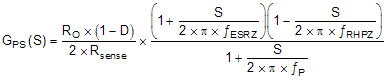where

• D is the switching duty cycle
• RO is the output load resistance
• Rsense is the equivalent internal current sense resistor, which is 0.08 Ω
Equation 14.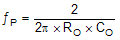where

• CO is output capacitor
Equation 15.where

• RESR is the equivalent series resistance of the output capacitor
Equation 16.The COMP pin is the output of the internal transconductance amplifier. Equation 17 shows the small signal transfer function of compensation network.

Equation 17.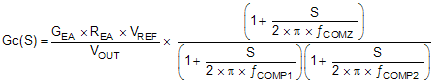where

• GEA is the transconductance of the amplifier
• REA is the output resistance of the amplifier
• VREF is the reference voltage at the FB pin
• VOUT is the output voltage
• ƒCOMP1, ƒCOMP2 are the poles' frequency of the compensation network
• ƒCOMZ is the zero's frequency of the compensation network

The next step is to choose the loop crossover frequency, ƒC. The higher in frequency that the loop gain stays above zero before crossing over, the faster the loop response is. It is generally accepted that the loop gain cross over no higher than the lower of either 1/10 of the switching frequency, ƒSW, or 1/5 of the RHPZ frequency, ƒRHPZ.

Then set the value of R5, C5, and C8 (in Figure 8-1) by following these equations.

Equation 18.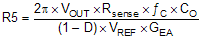where

• ƒC is the selected crossover frequency

The value of C5 can be set by Equation 19.

Equation 19.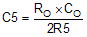The value of C8 can be set by Equation 20.

Equation 20.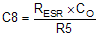If the calculated value of C8 is less than 10 pF, it can be left open.

Designing the loop for greater than 45° of phase margin and greater than 10-dB gain margin eliminates output voltage ringing during the line and load transient.